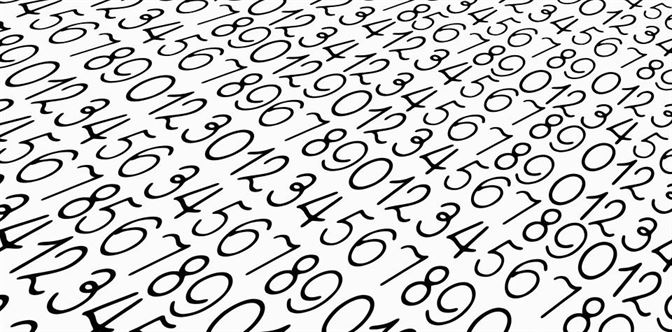# Error Arithmetic Operations Of Significant Figures

##The number of significant figures in a result is simply the number of figures that are known with some degree of reliability. The number 13.2 is said to have 3 significant figures. Non-zero digits are always significant. 3.14159 has six significant digits (all the numbers give you useful information). Thus, 67 has two significant digits, and 67.3 has three significant digits. In this article, we will learn about the rules for arithmetic operations of significant figures.

## What are Significant Figures?

Significant figures can be defined as important numbers from 0 to 9 that are used in the coefficient of an expression that explains the precision of the expression. These figures are obtained by rounding off the expression after the calculations are executed on them. There are certain rules to follow when it comes to arithmetic operations. Following is the table of examples with decimal notation, scientific notation, and significant figures:

 Decimal notation Scientific notation Significant figures 1,324,000.00 1.324*106 4 1.32400000*106 9 0.00006750000 6.75*10-5 3 6.750000*10-5 7 -0.0000000100 -1*10-8 1 -1.00*10-8 3

## Rules for Arithmetic Operation of Significant Figures

The general rule to follow is the rule of the weakest link which states that the chain is as strong as its weakest link. The accuracy of a measurement or a calculation is limited by the least accurate measurement in the calculation.

Following is the set of arithmetic operations that are used in significant figures are:

• Subtraction
• Multiplication
• Division

Following is the table with the link related to significant figures:

The result is rounded off to the last common digit occurring furthest to the right in all components. Another way to state this rule is as follows: in addition and subtraction, the result is rounded off so that it has the same number of digits as the measurement having the fewest decimal places (counting from left to right). For example,

200 (assume 3 significant figures) + 69.693 (5 significant figures) = 269.693

Which should be rounded to 269 (3 significant figures). Note, however, that it is possible two numbers have no common digits (significant figures in the same digit column).

13.214 + 234.6 + 7.0350 + 6.38 = 261.2290

We can see that the second number, 234.6, is only accurate to the tenths place; all the other numbers are accurate to a greater number of decimal places. Complying with the rule of the weakest link, the answer should be rounded to the tenth place. We get,

13.214 + 234.6 + 7.0350 + 6.38 = 261.2

### Multiplication and Division

Let’s see how to perform multiplication and division by keeping significant figures. The multiplication is performed normally and the decimal points are all noted down. The rule of the weakest link also extends to the multiplication of significant figures. In both multiplication and division, the result should be rounded off to have the same number of significant figures as the component with the least number of significant figures.

16.235 × 0.217 × 5 = 17.614975

Since the component with the lowest number of significant digits has only one significant digit, we will need to round 17.614975 to 20 which is the nearest answer with one significant digit. Another example is,

0.00435 × 4.6 = 0.02001

4.6 here has only 2 significant digits, we will round 0.02001 to two significant digits. From this, we get,

0.00435 × 4.6 = 0.020

0.02 here is not the right answer, because 0.02 has only one significant digit; namely, the “2”. The trailing zero in 0.020 indicates that “this is accurate to the thousandths place, or two significant digits”, and is necessary to complete the answer.## Frequently Asked Questions – FAQs

Q1

### What are significant figures ?

Significant figures can be defined as important numbers between 0 and 9 that are used in the coefficient of an expression that explains the precision of the expression. These figures are obtained by rounding off the expression after the calculations are executed on them.
Q2

### What are the set of arithmetic operations that are used in significant figures?

Following is the set of arithmetic operations that are used in significant figures are:
• Subtraction
• Multiplication
• Division
Q3

### What is rounding off?

Rounding off means a number is made simpler by keeping its value intact but closer to the next number. It is done for whole numbers, and for decimals at various places of hundreds, tens, tenths, etc. Rounding off numbers is done to preserve the significant figures.
Q4

### What are the dimensions of a physical quantity?

Dimensions of a physical quantity refer to the nature of the quantity which defines it in some measurable terms. The physical dimensions are expressed in terms of length, mass and time represented by L, M and T respectively.
Q5

### Define supplementary units.

Supplementary units are dimensionless physical quantities that are used along with fundamental units.

## Watch the video and understand Error and Error PropagationTest your Knowledge on Error Arithmetic Operations Of Significant Figures